# Numpy基础入门（7）IO文件操作以及Matplotlib库的使用

## NumPy - IO

ndarray对象可以保存到磁盘文件并从磁盘文件加载。 可用的 IO 功能有：

NumPy 为ndarray对象引入了一个简单的文件格式。 这个npy文件在磁盘文件中，存储重建ndarray所需的数据、图形、dtype和其他信息，以便正确获取数组，即使该文件在具有不同架构的另一台机器上。

1.numpy.save()

numpy.save()文件将输入数组存储在具有npy扩展名的磁盘文件中。

>>>import numpy as np
>>>a = np.array([1,2,3,4,5])
>>>np.save('outfile',a)


>>>import numpy as np
>>>b
array([1, 2, 3, 4, 5])


2.savetxt()

>>>import numpy as np
>>>a = np.array([1,2,3,4,5])
>>>np.savetxt('out.txt',a)
>>>b
array[ 1.  2.  3.  4.  5.]


## Matplotlib 绘制直方图

NumPy 有一个numpy.histogram()函数，它是数据的频率分布的图形表示。 水平尺寸相等的矩形对应于类间隔，称为bin，变量height对应于频率。

1.numpy.histogram()

numpy.histogram()函数将输入数组和bin作为两个参数。 bin数组中的连续元素用作每个bin的边界。


>>>import numpy as np
>>>a = np.array([22,87,5,43,56,73,55,54,11,20,51,5,79,31,27]) ]
>>>np.histogram(a,bins =  [0,20,40,60,80,100])
>>>hist,bins = np.histogram(a,bins =  [0,20,40,60,80,100])
>>>hist
array([3, 4, 5, 2, 1], dtype=int64)

>>>bins
array([  0,  20,  40,  60,  80, 100])


2.plt()

Matplotlib 可以将直方图的数字表示转换为图形。 pyplot子模块的plt()函数将包含数据和bin数组的数组作为参数，并转换为直方图。

>>>from matplotlib import pyplot as plt
>>>import numpy as np
>>>a = np.array([22,87,5,43,56,73,55,54,11,20,51,5,79,31,27])
>>>plt.hist(a, bins=[0,20,40,60,80,100])
>>>plt.title("histogram")
>>>plt.show()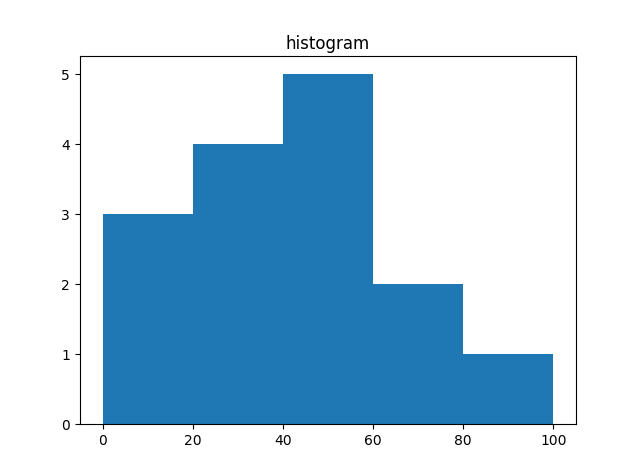3.pyplot()

pyplot()是 matplotlib 库中最重要的函数，用于绘制 2D 数据。

>>>import numpy as np
>>>from matplotlib import pyplot as plt
>>>x = np.arange(1,11)
>>>y =  2  * x +  5
>>>plt.title("Matplotlib demo")
>>>plt.xlabel("x axis caption")
>>>plt.ylabel("y axis caption")
>>>plt.plot(x,y)
>>>plt.show()


ndarray对象x由np.arange()函数创建为x轴上的值。y轴上的对应值存储在另一个数组对象y中。 这些值使用matplotlib软件包的pyplot子模块的plot()函数绘制。图形由show()函数展示。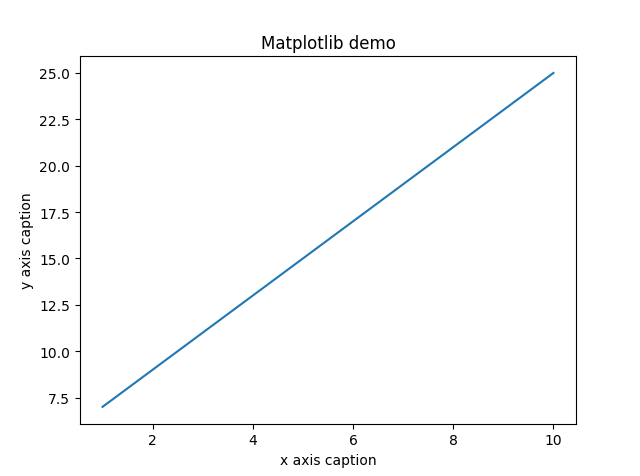‘-’ 实线样式
‘–’ 短横线样式
‘-.’ 点划线样式
‘:’ 虚线样式
‘,’ 像素标记
‘o’ 圆标记
‘v’ 倒三角标记
‘^’ 正三角标记
‘<’ 左三角标记
‘>’ 右三角标记
‘1’ 下箭头标记
‘2’ 上箭头标记
‘3’ 左箭头标记
‘4’ 右箭头标记
‘s’ 正方形标记
‘p’ 五边形标记
‘*’ 星形标记
‘h’ 六边形标记 1
‘H’ 六边形标记 2
‘+’ 加号标记
‘x’ X 标记
‘D’ 菱形标记
‘d’ 窄菱形标记
‘|’ 竖直线标记
‘_’ 水平线标记

‘b’ 蓝色
‘g’ 绿色
‘r’ 红色
‘c’ 青色
‘m’ 品红色
‘y’ 黄色
‘k’ 黑色
‘w’ 白色

>>>import numpy as np
>>>from matplotlib import pyplot as plt
>>>x = np.arange(1,11)
>>>y =  2  * x +  5
>>>plt.title("Matplotlib demo")
>>>plt.xlabel("x axis caption")
>>>plt.ylabel("y axis caption")
>>>plt.plot(x,y,"ob")
>>>plt.show()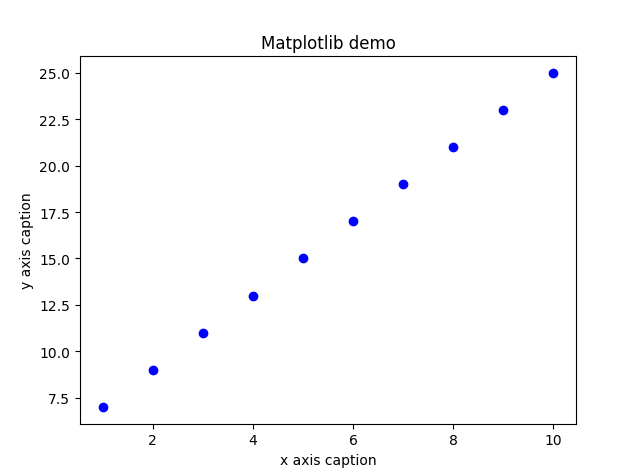4.绘制正弦波

>>>import numpy as np
>>>import matplotlib.pyplot as plt
# 计算正弦曲线上点的 x 和 y 坐标
>>>x = np.arange(0,  3  * np.pi,  0.1)
>>>y = np.sin(x)
>>>plt.title("sine wave form")
# 使用 matplotlib 来绘制点
>>>plt.plot(x, y)
>>>plt.show()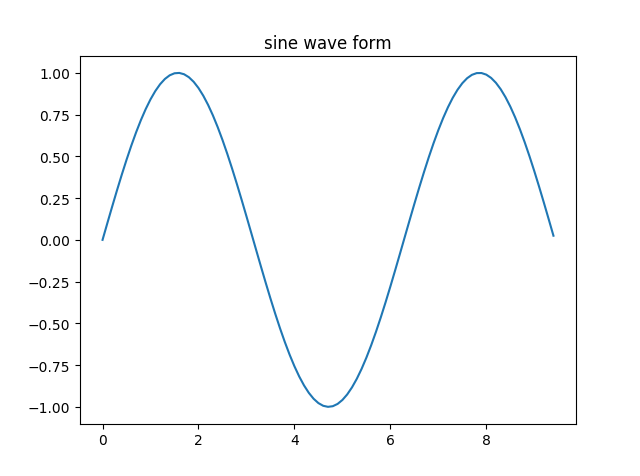5.subplot()

subplot()函数允许你在同一图中绘制不同的东西。 在下面的脚本中，绘制正弦和余弦值。

>>>import numpy as np
>>>import matplotlib.pyplot as plt
# 计算正弦和余弦曲线上的点的 x 和 y 坐标
>>>x = np.arange(0,  3  * np.pi,  0.1)
>>>y_sin = np.sin(x)
>>>y_cos = np.cos(x)
# 建立 subplot 网格，高为 2，宽为 1
# 激活第一个 subplot
>>>plt.subplot(2,  1,  1)
# 绘制第一个图像
>>>plt.plot(x, y_sin)
>>>plt.title('Sine')
# 将第二个 subplot 激活，并绘制第二个图像
>>>plt.subplot(2,  1,  2)
>>>plt.plot(x, y_cos)
>>>plt.title('Cosine')
# 展示图像
>>>plt.show()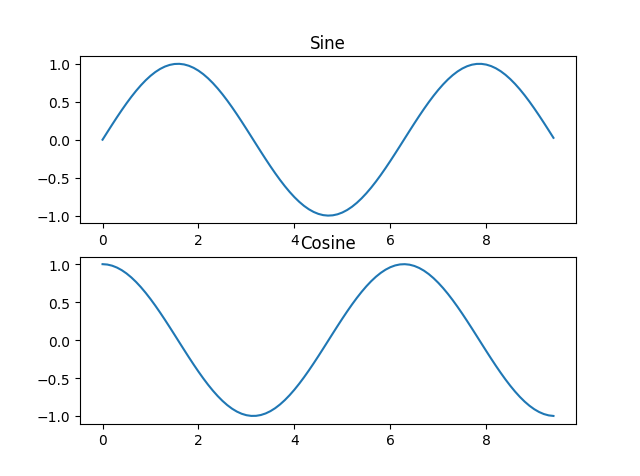6.bar()

pyplot子模块提供bar()函数来生成条形图。 以下示例生成两组x和y数组的条形图。

>>>from matplotlib import pyplot as plt
>>>x =  [5,8,10]
>>>y =  [12,16,6]
>>>x2 =  [6,9,11]
>>>y2 =  [6,15,7]
>>>plt.bar(x, y, align =  'center')
>>>plt.bar(x2, y2, color =  'g', align =  'center')
>>>plt.title('Bar graph')
>>>plt.ylabel('Y axis')
>>>plt.xlabel('X axis')
>>>plt.show()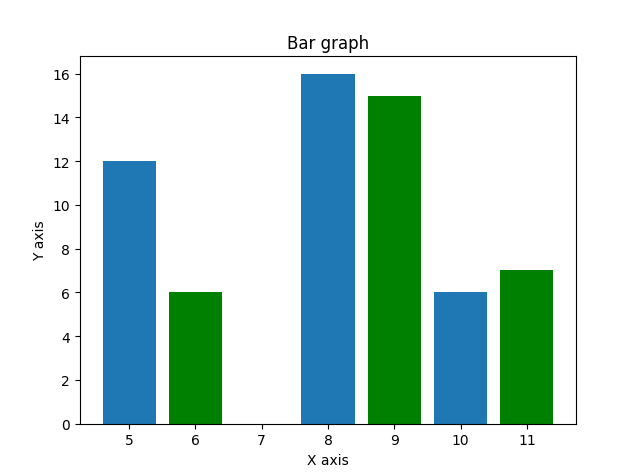©️2019 CSDN 皮肤主题: 大白 设计师: CSDN官方博客